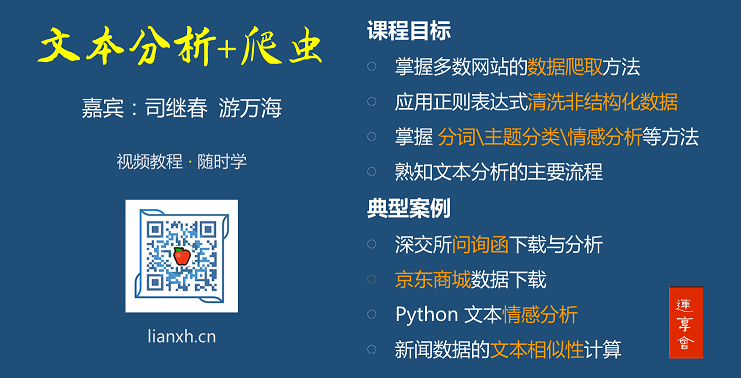# Stata: 断点回归 (RDD) 中的平滑性检验

E-mail: 1016201399@qq.com

Stata连享会   主页 || 视频 || 推文## 2. 检验工具

Note: 后面分析需要使用 `rdrobust` 命令对应的相关程序和数据，请执行如下两条命令下载外部命令 (默认存储于 ..\ado\plus 文件夹下) 和相关数据 (默认存储于当前工作路径下，下载完成后输入 `cd` 可以查看当前路径地址；输入 `dir` 可以查看当前工作路径下的文件)：

• ounter(line
• ounter(line
``. net install st0366_1.pkg, replace //rdrobust外部命令``. net get     st0366_1.pkg, replace //rdrobust相关数据``

### 2.1 使用 histogram 命令

• 优点： 其操作简单、直观。
• 缺点： 直方图在不同组间距中的样本数量的不同，使得很难直观上观测到连续性。
• ounter(line
• ounter(line
• ounter(line
• ounter(line
• ounter(line
• ounter(line
• ounter(line
• ounter(line
• ounter(line
• ounter(line
• ounter(line
``*----------使用直方图显示分配变量的连续性 figure2 ------``*-使用 David S.Lee (2007) 参议院选举的数据`` use "https://gitee.com/arlionn/data/raw/master/data01/rdrobust_rdsenate.dta", clear`` *use "rdrobust_senate.dta", clear  `` #d ;``  histogram margin, ``    lcolor(brown) fcolor(gs16) ``    title("Senate_selection")  ``    xtitle("margin") ``    note("figure2");`` #d cr``

### 2.2 使用 McGrary 方法

`DCdensity` 命令是由 McCrary (2006) 提出来用于检验分配变量在临界值处是否连续，即样本在临界值处是否存在人为干预现象。主要方法如下：

• (1) 生成分配变量的密度直方图，确保临界值不会被直方图所覆盖；
• (2) 在临界值左右两侧，分别进行局部的线性回归。其中因变量是组内频数，自变量是组间距的中间值；
• (3) 检验临界值两侧的拟合值的差的对数值是否显著不为零。

• 该命令相对于绘制直方图，绘制出较为平滑的核密度曲线图以及置信区间，能够直观的现实分配变量在断点处的连续性。

• (1) 该命令所使用的核密度函数的带宽 (bandwith) 并不是使用交互验证或者是插入法确定的最优带宽选择，而是由其编写的默认公式所确定，因此在整体断点回归中使用不同带宽无法有效地证明其分配变量的连续性。
• (2) 如果存在某种干预模式使得高于临界值点和低于临界值点处的样本数量相同，那么该命令无法有效识别该类分配变量的跳跃。
• (3) 图形无法进行注释修饰。

McGrary (2006) 分配变量密度函数连续检验的基本命令为 `DCdensity` , 其基本语法格式如下：

• ounter(line
`` DCdensity varlist [if] [in] [weight] [, options]``

• `varlist`: 分配变量
• `breakpoint()`: 分配变量临界值处
• `b()`： 指定特定带宽值
• `generate()`: 生成新的参数变量
• `graphname()`: 保存图形

• ounter(line
• ounter(line
• ounter(line
• ounter(line
• ounter(line
• ounter(line
• ounter(line
• ounter(line
• ounter(line
• ounter(line
``*----------使用 McGray (2006) 命令 figure3 ----------------``. DCdensity margin, breakpoint(0) gen(Xj Yj r0 fhat se_fhat)``  Discontinuity estimate (log difference in height): -.100745626``                                                   (.117145041)    ``. return list``  scalars:``          r(bandwidth) =  25.84938346120713``            r(binsize) =  1.841330210610218``                 r(se) =  .117145040900551``              r(theta) =  -.1007456257891786``

#### 2.3 使用 rdcont 命令

`rdcont` 命令的基本语法格式如下：

• ounter(line
`` rdcont running_var [if] [in], [options]``

• `running_var`: 分配变量
• `alpha()`: 指定用于计算最佳带宽的临界值
• `threshold()`: 指定测试的临界值
• `qband()`: 指定特定的带宽值

Stata 范例：

• ounter(line
• ounter(line
• ounter(line
• ounter(line
• ounter(line
• ounter(line
• ounter(line
• ounter(line
• ounter(line
• ounter(line
• ounter(line
``*----------使用 rdcont 命令 table1 ----------------------``. rdcont margin, threshold(0)``RDD non-randomized approximate sign test``Running variable: margin``Cutoff c =       0 | Left of c  Right of c    Number of obverse = 1390``-------------------+----------------------                    q =   94``     Number of obs |       640         750``Eff. number of obs |        46          48`` Eff. neighborhood |    -1.743       1.824``-------------------+----------------------``           p-value |     0.918``

## 4. 参考资料

(1) Lee D S. Randomized experiments from non-random selection in US House elections[J]. Journal of Econometrics, 2008, 142(2): 675-697.[pdf]

(2) McCrary J. Manipulation of the running variable in the regression discontinuity design: A density test[J]. Journal of econometrics, 2008, 142(2): 698-714.[pdf]

(3) Bugni F A, Canay I A. Testing Continuity of a Density via g-order statistics in the Regression Discontinuity Design[J]. arXiv preprint arXiv:1803.07951, 2018.[pdf]

## 附：本文涉及的 Stata 代码

• ounter(line
• ounter(line
• ounter(line
• ounter(line
• ounter(line
• ounter(line
• ounter(line
• ounter(line
• ounter(line
• ounter(line
• ounter(line
• ounter(line
• ounter(line
• ounter(line
• ounter(line
• ounter(line
• ounter(line
• ounter(line
• ounter(line
• ounter(line
• ounter(line
• ounter(line
• ounter(line
• ounter(line
• ounter(line
• ounter(line
• ounter(line
• ounter(line
• ounter(line
• ounter(line
• ounter(line
• ounter(line
• ounter(line
• ounter(line
• ounter(line
• ounter(line
• ounter(line
• ounter(line
• ounter(line
• ounter(line
• ounter(line
• ounter(line
• ounter(line
• ounter(line
• ounter(line
• ounter(line
• ounter(line
• ounter(line
• ounter(line
• ounter(line
• ounter(line
• ounter(line
• ounter(line
• ounter(line
• ounter(line
``*-------驱动变量不连续图形figure1--------``*----生成模拟数据---`` clear`` set obs 5000`` set seed 123`` gen z = rnormal()*0.5       `` save simu1.dta, replace`` ``*----生成干预数据----   `` gen z0 = z if (z>=-0.15)&(z<0)`` gen z1 = z if (z>=0)&(z<0.15)    `` gen z2 = z`` replace z2 = . if z2 == z0`` stack z2 z1, into(z3) clear`` drop if z3 == . ``                       `` *-stack命令会将z变量删除，该命令将z变量添加进来`` merge 1:1 _n using simu1.dta, nogenerate`` drop if z3 == .``*-为了图形显示更加直观，进行轻微调整`` gen zNo  = z3 + 0.1`` gen zYes =  z - 0.1 	 `` `` #d ;``  twoway (histogram zYes,``	    frequency lcolor(gs12) fcolor(gs12)) ``	 (histogram zNo, ``	    frequency lcolor(black) fcolor(none)),``	  xline(0, lpattern(dash) lcolor(green) lwidth(*1.5))``	  xtitle("Score")``	  xlabel(-2 "40" -1 "50" 0 "60" 1 "80" 2 "90")``	  legend(label(1 "figure1(a) 无人为干预")``	         label(2 "figure1(b) 人为干预"))``	  note("figure1")``	  scheme(burd);`` #d cr`` ``*----------使用直方图显示分配变量的连续性figure2------`` * use "rdrobust_senate.dta", clear`` use https://gitee.com/arlionn/data/raw/master/data01/rdrobust_rdsenate.dta, clear`` #d ;``  histogram margin, ``     lcolor(brown) fcolor(gs16) ``     title("Senate_selection")  ``  	xtitle("margin") `` 	note("figure2");`` #d cr`` ``*----------使用McGray(2006)命令figure3----------------`` DCdensity margin, breakpoint(0) gen(Xj Yj r0 fhat se_fhat)`` return list``	``*----------使用rdcont命令table1----------------------`` rdcont margin, threshold(0)``

http://lianxh.duanshu.com

#### 连享会 - 文本分析与爬虫 - 专题视频#### 关于我们

• Stata连享会 由中山大学连玉君老师团队创办，定期分享实证分析经验。直播间 有很多视频课程，可以随时观看。
• 连享会-主页知乎专栏，300+ 推文，实证分析不再抓狂。
• 公众号推文分类： 计量专题 | 分类推文 | 资源工具。推文分成 内生性 | 空间计量 | 时序面板 | 结果输出 | 交乘调节 五类，主流方法介绍一目了然：DID, RDD, IV, GMM, FE, Probit 等。
• 公众号关键词搜索/回复 功能已经上线。大家可以在公众号左下角点击键盘图标，输入简要关键词，以便快速呈现历史推文，获取工具软件和数据下载。常见关键词：
• `课程, 直播, 视频, 客服, 模型设定, 研究设计, 暑期班`
• `stata, plus，Profile, 手册, SJ, 外部命令, profile, mata, 绘图, 编程, 数据, 可视化`
• `DID，RDD, PSM，IV，DID, DDD, 合成控制法，内生性, 事件研究`, `交乘, 平方项, 缺失值, 离群值, 缩尾, R2, 乱码, 结果`
• `Probit, Logit, tobit, MLE, GMM, DEA, Bootstrap, bs, MC, TFP`, `面板, 直击面板数据, 动态面板, VAR, 生存分析, 分位数`
• `空间, 空间计量, 连老师, 直播, 爬虫, 文本, 正则, python`
• `Markdown, Markdown幻灯片, marp, 工具, 软件, Sai2, gInk, Annotator, 手写批注`, `盈余管理, 特斯拉, 甲壳虫, 论文重现`, `易懂教程, 码云, 教程, 知乎`